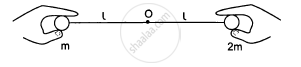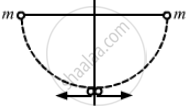Advertisement Remove all ads

# Two Balls Having Masses M and 2m Are Fastened to Two Light Strings of Same Length L. the Other Ends of the Strings Are Fixed at O. the Strings Are - Physics

Sum

Two balls having masses m and 2m are fastened to two light strings of same length l (See figure). The other ends of the strings are fixed at O. The strings are kept in the same horizontal line and the system is released from rest. The collision between the balls is elastic. (a) Find the velocity of the balls just after their collision. (b) How high will the ball rise after the collision?Advertisement Remove all ads

#### Solution

Given:
Mass of the 1st ball = m
Mass of the 2nd ball = 2m

When the balls reach the lower end,
Let the velocity of m be v; and the velocity of 2m be v'.

Using the work-energy theorem, we can write:

$\left( \frac{1}{2} \right) \times m v^2 - \left( \frac{1}{2} \right) \times m(0 )^2 = mgl$

$\Rightarrow v = \sqrt{2gl}$

$\text{ Similarly, velocity of block having mass 2m will be, }$

$v' = \sqrt{2gl}$

$\therefore v = v' = u (say)$
Let the velocities of m and 2m after the collision be v1 and v2 respectively.Using the law of conservation of momentum, we can write:

$m \times u - 2mu = m v_1 + 2m v_2$

$\Rightarrow v_1 + 2 v_2 = - u . . . (1)$

$\text{ On applying collision formula, we get: }$

$v_1 - v_2 = [(u - v)] = - 2u . . . (2)$

$\text{ Substracting equation } \left( 1 \right) \text{ from } \left( 2 \right), \text{ we get: }$

$3 v_2 = u$

$\Rightarrow v_2 = \frac{u}{3} = \sqrt{\frac{2gl}{3}}$

$\text{ Substituting this value in equation (1), we get: }$

$v_1 - v_2 = - 2u$

$\Rightarrow v_1 = - 2u + v_2$

$= - 2u + \left( \frac{u}{3} \right)$

$= \frac{- 5}{3}u = \frac{- 5}{3} \times \sqrt{2gl}$

$= \frac{- \sqrt{50gl}}{3}$

(b) Let the heights reached by balls 2m and m be h1 and h respectively.

Using the work energy principle, we get:

$\left( \frac{1}{2} \right) \times 2m \times (0 )^2 - \left( \frac{1}{2} \right) \times 2m \times \left( v_2 \right)^2 = - 2m \times g \times h_1$

$\Rightarrow h_1 = \left( \frac{l}{9} \right)$

$\text{ Similarly,}$

$\left( \frac{1}{2} \right) \times m \times (0 )^2 - \left( \frac{1}{2} \right) \times m v_1^2 = m \times g \times h_2$

$\Rightarrow \left( \frac{1}{2} \right) \times \frac{50gl}{9} = g \times h_2$

$\Rightarrow h_2 = \frac{25l}{9}$
As h2 is more than 2l, the velocity at the highest point will not be zero.
Also, the mass m will rise by a distance 2l.

Is there an error in this question or solution?
Advertisement Remove all ads

#### APPEARS IN

HC Verma Class 11, 12 Concepts of Physics 1
Chapter 9 Centre of Mass, Linear Momentum, Collision
Q 56 | Page 163
Advertisement Remove all ads
Advertisement Remove all ads
Share
Notifications

View all notifications

Forgot password?